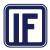# Problem after having set the dropdown value

GridTop achievementsRank 2
Michele asked on 29 Aug 2013, 09:36 AM
Hello,
I've a kendo UI grid that has a column with editortemplate....
He're the grid

`@(Html.Kendo()`
`    ``.Grid<``DO.Admin.Utente``>()`
`    ``.Name("grid")`
`    ``.Columns(columns =>`
`    ``{`
`        ``columns.Bound(x => x.IDDipendente).Visible(false);`
`        ``columns.Bound(x => x.IDUtente);`
`        ``columns.Bound(x => x.Nominativo);`
`        ``columns.Bound(x => x.Societa);`
`        ``columns.Bound(x => x.Filiale);`
`        ``columns.Bound(x => x.Ruolo);`
`        ``columns.Bound(x => x.Profilo);`
`        ``columns.Bound(x => x.Funzioni);`
`        ``columns.Bound(x => x.Stato).EditorTemplateName("StatoTemplate");//ClientTemplate("#=stati.statiName#");`
`        ``columns.Bound(x => x.AccessoEureka);`

`    ``})`
`    ``.Pageable()`
`    ``.Scrollable()`
`    ``.Editable(editable => editable.Mode(GridEditMode.InCell))`
`    ``.DataSource(dataSource => dataSource`
`        ``.Ajax()`
`        ``.Model(model => `
`        ``{`
`            ``model.Id(x => x.IDDipendente);`
`            ``model.Field(x => x.IDUtente).Editable(false);`
`            ``model.Field(x => x.Nominativo).Editable(false);`
`            ``model.Field(x => x.Societa).Editable(false);`
`            ``model.Field(x => x.Filiale);`
`            ``model.Field(x => x.Ruolo);`
`            ``model.Field(x => x.Profilo);`
`            ``model.Field(x => x.Funzioni);`
`            ``model.Field(x => x.Stato);`
`            ``model.Field(x => x.AccessoEureka);`
`                `
`        ``})`
`        ``.Read(read => read.Action("GetListaUtenti", "GestioneUtenti"))`
`    ``)`
`)`

And here's the editor template
`@{`
`    ``Layout = null;`
`}`

`@(Html.Kendo().DropDownList()`
`          ``.Name("Stato")`
`          ``.HtmlAttributes(new { style = "width: 250px" })`
`          ``.DataTextField("Descr")`
`          ``.DataValueField("ID")`
`          ``.DataSource(source =>`
`          ``{`
`              ``source.Read(read =>`
`              ``{`
`                  ``read.Action("GetListaStati", "Common", new { Area = string.Empty });`
`              ``}).ServerFiltering(false);`
`          ``})`
`    ``)`

Consider the List of Stato items
as

0 | Active
1 | Disactive

when I show the grid in read I've my columns that shows Active/Disactive based on a field called Stato that's string....
After I change the dropdown I got 0/1 instead.... how do I fix this?

Second question, in my model I also have a IdStato that's 0/1 and its used when updating the model.... should I put this as an Hidden column? how do I update that field as well?
Paolo

## 2 Answers, 1 is accepted

0Alexander Popov
Telerik teamanswered on 30 Aug 2013, 01:58 PM
Hi Paolo,

There is a similar scenario demonstrated in our offline examples located here:
• "Path_to_KendoUI\wrappers\aspnetmvc\Examples\Areas\razor\Views\web\grid\editing_custom.cshtml"
• "Path_to_KendoUI\\wrappers\aspnetmvc\Examples\Areas\razor\Views\web\grid\EditorTemplates\ClientCategory.cshtml"
Using the approach shown in the above example you will not need to include the IdStato column.
In case the provided information does not help you resolve the case please attach a runnable project where the issue is reproduced. This would help us pinpoint the exact reason for this behavior.

Regards,
Alexander Popov
Telerik
0Michele
Top achievementsRank 2
answered on 30 Aug 2013, 02:17 PM
Hello Alexander,
I've fixed using ForeignKey fields!
Thats' ok! Thanks
Tags
GridMichele
Top achievementsRank 2Rank 2